# High Performance Computing BE Computer (2012 Course) Endsem May 2016

Q1) a) What are applications of Parallel Computing?

1. i) The parallel computing is suitable for the problems require much more time for computation completion. The several areas in which parallel computing are used usually based on high-end engineering and scientfic problems.
2. ii) This includes computer based simulations and Computational Fluid Dynamics (CFD) as well as other computer based digital image processing and security algorithms.

1. A) Applications of parallel computing in Engineering.

1. i) Parallel computing is used in various engineering problems. These problems typically solved in the form of computer programs in traditional machines.
2. ii) These machines are capable of performing the tasks sequentially but designers closely observed and found the subtasks included in the problem can be performed as different processes simultaneously.

iii) The parallel computations provide the improvements in overall efficiency of the applicatiom. The main factor responsible for efficiency improvements is the speedup of the computations.

1. iv) This phenomenon is adopted in several engineering application domains such as applications of

aerodynamics, optimization algorithms like branch and bound and genetic programming etc.

1. B) Scientfic Applications using Parallel Computing.

1. i) In short scientfic applications are created to show the simulated behaviours of real world entities by using mathematics and mathematical formulas.
2. ii) This means the object exists in the real world are mapped in the form of mathematical models and actions present in that object are simulated using mathematical formula.

iii) Simulations are based on very high-end calculations and require the use of powerful computers. The most of the powerful computers are parallel computers and do the computations in parallel

form.

1. iv) The scientfic applications are major candidates for the parallel computations. For example weather forecasting and climate modeling, Oil exploration and energy research, Drug discovery and genomic research etc. are some of the scientfic applications in which parallel activities are carried out throughout the completion.
2. v) These activities are performed by the tools and techniques provided under the field of parallel computing.

1. C) Commercial Applications Based on Parallel Computing.

1. i) The increasing need for more processing power is full-filled by parallel architectures. The parallel architectures continuously evolve to satisfy the processing requirements of the developing trends of the society.
2. ii) The commercial applications require more processing power in the current market trends because of performing many activities simultaneously.

iii) This creates an inclination towards the use of parallel computing applications for commercial problems. We can consider multimedia applications for commercial problems. We can consider multimedia applications as an example of commercial applications in which parallel computing plays vital role.

1. iv) For large commercial applications such as multimedia applications enhance processing power and most of the circumstances the overall applications should be divided into different modules. These modules work in the close cooperation to complete the overall required task in less amount of time.

1. D) Parallel Computing Applications in Computer Systems.

1. i) In the recent developments in computing the techniques of parallel processing becomes easily adoptable because of growth in computer and communication networks.
2. ii) The computations perform in parallel, by the use of collection of low power computing devices in the form of clusters. In fact a group of computers configured to solve a problem by means of parallel processing is termed as cluster computing systems.

iii) The advances in cluster computing in terms of software frameworks made possible to utilize the computing power of multiple devices for solving of large computing tasks efficiently.

1. iv) The parallel computing is effectively applied in the field of computer security. The internet based parallel computing set-up is suitably used for the implementation of extremely large set of data required for computations.

Q1) b) Explain basic working Principal of VLIW Processor?

1. i) Very Long Instruction Word(VLIW) are one of the suitable options to achieve Instruction Level Parallelism in programs. This means VLIW architectures can run more than one instruction at a same time in a program.

1. ii) The following are some of the points can be considered as principles on which Very Long Instruction Word(VLIW) Processor works.
2. VLIW processor’s basic aim is to speeding up the computation by achieving Instruction Level Parallelism (ILP).
3. VLIW uses same hardware core as superscalar processors with multiple Execution Units (EUs)

working in parallel.

1. In VLIW Processor, an instruction consists of more than one operations in which typical word length is from 52 bits to 1 Kbits.
2. All the operations in the instruction are executed in a lock-step mode.
3. The VLIW Processor is dependent on compiler to find the parallelism and the scheduled dependency in the program code.

Q2) a) Explain Randomized Block Distribution and Hierarchical Mapping.

a.1) Randomized Block Distribution.

1. i) Randomized block distribution is a more general form of distribution. Here load balancing is achieved by dividing the array into more number of blocks than total the total number of process currently active for processing. i.e. Divide the data such that Number of blocks in which data is divided > Total Number of Active Processes.
2. ii) Load Balancing is the mechanism to divide the data such that each process will get equal amount of data to work on.

iii) As the name suggests, in this distribution blocks are distributed randomly among the processes but the block distribution is handled uniformly.

1. iv) Consider an example of one dimensional block distribution in randomized fashion.

V = {2,3,5,8,12,23,45,67,89,90,102,110}

1. v) Applying Random function on block

Random(V) = {67,12,2,90,45,8,110,3,67,23,5,102}

1. vi) Considering the block size as 3 so the number of processes will be 4.

(3*4  = 12(Total Number of elements)).

Vii) Therefore P0 = {67,12,2}, P1 = {90,45,8}, P3 = {110,3,67}, P4 = {23,5,102}

viii) This is how the technique of Randomized Block Distribution works.

a.2) Hierarchical Mapping.

1. i) Hierarchical mapping technique can be applied on those task that can be represented using task dependency graph. The mapping in this approach also has some problems like load-imbalance or inadequate graph.
2. ii) For Example lets take Hierarchical mapping for binary tree task dependency graph. One such tree is shown in the figure. Here at the top of the tree only few concurrent tasks can be performed because of availability of less number of tasks.

iii) The decomposition of tasks in done at further level where number of tasks are greater so that mapping can be handled efficiently. In such cases, large tasks are divided into smaller subtasks at further levels.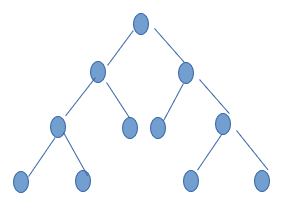1. iv) The above binary tree has 4 levels. Initially the root task can be divided and assigned to 4 prosesses. In similar fashion, the next level task can also be divided and assigned to 4 Processes.
2. v) These divisions at different levels are mainly used for mapping of tasks to processes and finally at leaf levels the tasks are mapped with processes as one to one mapping.

Q2) b) Write a note on: Topologies and Embedding.

1. i) The processses which are involved in the group are identified with their ranks. These processes can communicate with each other and their communication pattern is represented using graph.
2. ii) Nodes in the graph represents process and edge between them represents the communication. That means if two nodes are connected with an edge then there is a communication between two processes.

iii) Communicator is a group of processes and a communicator consists of many attributes. Topology is one of them.

1. iv) Topology is considered as an additional information and can be associated with the communicator beyond with the groups and context. This important additional inforrmation is said to be cached with communicator.
2. iv) MPI supports mainly creattion of two types of topologies
3. Cartesian Topology.

2.Graph Topology.

1. v) The graph is a collection of nodes and edges. In the graph based topology, processes are represented as nodes and edges are used to show connection between them.
2. vi) Grid based topology creates a ring , two dimensional and three dimensional grids, or tori etc.

1. Cartesian Topology.

1. i) The processes are connected with each other in a virtual grid like fashion. This type of topologies is suitable for grid like structures.
2. ii) While programming using MPI, the grid like structure is easier than graph based topology. A row major numbering system is used for mapping of the grid structures.Fig: 2*2 Grid

iii) In Cartesian Topology process coordinates begin with 0 and it supports Row-major Numbering.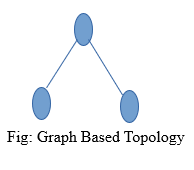1. i) In this type of topology, Nodes represents the Process and the edge between two nodes represents the communication between them.

Q3) a) Implement Producer Consumer Problem using Matrix Synchronization Primitives in pthreads.

Basically, the producer produces goods while the consumer consumes the goods and typically does something with them.

In our case our producer will produce an item and place it in a bound-buffer for the consumer. Then the consumer will remove the item from the buffer and print it to the screen.

A buffer is a container of sorts; a bound-buffer is a container with a limit. We have to be very careful in our case that we don’t over fill the buffer or remove something that isn’t there; in c this will produce a segmentation fault.

What: A synchronization tool used in concurrent programming

Where: We will use semaphores in any place where we may have concurrency issues. In other words any place where we feel more than one thread will access the data or structure at any given time.

When: To help solve concurrency issues when programming with threads.

Why: Think about how registers work in the operating system for a second. Here is an example of how registers work when you increment a counter-

register1 = counter;

register1 = register1 + 1;

counter = register1;

Now image two threads manipulating this same example but one thread is decrementing–

(T1) register1 = counter; [register1 = 5]

(T1) register1 = register1 + 1;[register1 = 6]

(T2) register2 = counter; [register2 = 5]

(T2) register2 = register2 – 1; [register2 = 4]

(T1) counter = register1; [counter = 6]

(T2) counter = register2;[counter = 4]

Because both threads were allowed to run without synchronization our counter now has a definitely wrong value. With synchronization the answer should come out to be 5 like it started.

How: We implement a semaphore as an integer value that is only accessible through two atomic operations wait() and signal(). Defined as follows:

/* The wait operation */

wait(S) {

while(S <= 0); //no-operation

S–;

}

/* The signal operation */

signal(S) {

S++;

}

S: Semaphore

The operation wait() tells the system that we are about to enter a critical section and signal() notifies that we have left the critical section and it is now accessible to other threads.

Therefore:

wait(mutex);

//critical section where the work is done

signal(mutex)

//continue on with life

Mutex stands for mutual exclusion. Meaning only one process may execute the section at a time.

We have an example that demonstrates how semaphores are used in reference to pthreads coming up right after this problem walk-through.

Basically, we are going to have a program that creates an N number of producer and consumer threads. The job of the producer will be to generate a random number and place it in a bound-buffer. The role of the consumer will be to remove items from the bound-buffer and print them to the screen. Remember the big issue here is concurrency so we will be using semaphores to help prevent any issues that might occur. To double our efforts we will also be using a pthread mutex lock to further guarantee synchronization.

The user will pass in three arguments to start to application: <INT, time for the main method to sleep before termination> <INT, Number of producer threads> <INT, number of consumer threads>

We will then use a function in initialize the data, semaphores, mutex lock, and pthread attributes.

Put main() to sleep().

Exit the program

Q3) b) Describe Barrier Synchronization for Shared Address Space Model.

1. i) In MPI programming, point to point communication is handled using message passing whereas global synchronization is done using collective communications.
2. ii) Important point while using communication is synchronization point among processes.

iii) MPI provides special function which is designed and implemented for synchronization named as MPI_Barrier().

1. iv) The working of this function is such a way that no process a allowed to cross a barrier untill all the processes have reached up to that barrier in their respective codes.
2. v) Syntax: int MPI_Barrier(MPI_Communication)
3. vi) The argument passed to this function is the communicator. A group of processes need to be synchronized are defined in communicator. Calling process blocks until all the processes in the given communicator have called it. This means the call only returns when all processes have entered the call.

MPI_Barrier()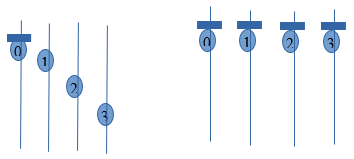vii) The MPI_Barrier() function is invoked by process 0.

viii) When Process 0 reaches barrier, it stops and wait for remaining process to reach to the barrier point.

1. ix) After every process reaches barrier point, the execution continues. Like this synchronization is achieved using Barrier.

Q3) a) Describe Logical Memory Model of a Thread?

Consider the following code segment that computes the product of two dense matrices of size n x n.

1   for (row = 0; row < n; row++)

2       for (column = 0; column < n; column++)

3           c[row][column] =

4               dot_product(get_row(a, row),

5                           get_col(b, col));

The for loop in this code fragment has n2 iterations, each of which can be executed independently. Such an independent sequence of instructions is referred to as a thread. In the example presented above, there are n2 threads, one for each iteration of the for-loop. Since each of these threads can be executed independently of the others, they can be scheduled concurrently on multiple processors. We can transform the above code segment as follows:

1   for (row = 0; row < n; row++)

2       for (column = 0; column < n; column++)

3           c[row][column] =

5                                         get_col(b, col)));

Here, we use a function, create_thread, to provide a mechanism for specifying a C function as a thread. The underlying system can then schedule these threads on multiple processors.While this logical machine model gives the view of an equally accessible address space, physical realizations of this model deviate from this assumption. In distributed shared address space machines such as the Origin 2000, the cost to access a physically local memory may be an order of magnitude less than that of accessing remote memory. Even in architectures where the memory is truly equally accessible to all processors (such as shared bus architectures with global shared memory), the presence of caches with processors skews memory access time. Issues of locality of memory reference become important for extracting performance from such architectures.

Q4) b) Why Synchronization is important? Enlist Thread API’s for Mutex Synchronization.

1. i) Synchronization is the facility provided to the user in almost all programming models used in designing of parallel programs to control the ordering of events on different processors.
2. ii) The parallel executions of some programs depend on the synchronization because without using the synchronization these programs may produce wrong results. The demerit of the synchronization is expensiveness.

Why it is important?

With respect to multithreading, synchronization has the capability to control the access of multiple threads to shared resources. Without synchronization, it is possible for one thread to modify a shared object while another thread is in the process of using or updating that object’s value. This often leads to significant errors.

Thread synchronization is required whenever two threads share a resource or need to be aware of what the other threads in a process are doing. Mutexes are the most simple and primitive object used for the co-operative mutual exclusion required to share and protect resources. One thread owns a mutex by locking it successfully, when another thread tries to lock the mutex, that thread will not be allowed to successfully lock the mutex until the owner unlocks it. The mutex support provides different types and behaviors for mutexes that can be tuned to your application requirements.

The Mutex synchronization APIs are:

• pthread_lock_global_np() (Lock Global Mutex): locks a global mutex provided by the pthreads run-time.
• pthread_mutexattr_destroy() (Destroy Mutex Attributes Object): destroys a mutex attributes object and allows the system to reclaim any resources associated with that mutex attributes object.
• pthread_mutexattr_getkind_np() (Get Mutex Kind Attribute) : retrieves the kind attribute from the mutex attributes object specified by attr.
• pthread_mutexattr_getname_np() (Get Name from Mutex Attributes Object): retrieves the name attribute associated with the mutex attribute specified by attr.
• pthread_mutexattr_getpshared() (Get Process Shared Attribute from Mutex Attributes Object): retrieves the current setting of the process shared attribute from the mutex attributes object.
• pthread_mutexattr_gettype() (Get Mutex Type Attribute) : retrieves the type attribute from the mutex attributes object specified by attr.
• pthread_mutexattr_init() (Initialize Mutex Attributes Object): initializes the mutex attributes object referenced by attr to the default attributes.
• pthread_mutexattr_setkind_np() (Set Mutex Kind Attribute): sets the kind attribute in the mutex attributes object specified by attr.
• pthread_mutexattr_setname_np() (Set Name in Mutex Attributes Object): changes the name attribute associated with the mutex attribute specified by attr.
• pthread_mutexattr_setpshared() (Set Process Shared Attribute in Mutex Attributes Object): sets the current pshared attribute for the mutex attributes object.
• pthread_mutexattr_settype() (Set Mutex Type Attribute) sets the type attribute in the mutex attributes object specified by attr.
• pthread_mutex_destroy() (Destroy Mutex) :destroys the named mutex.
• pthread_mutex_getname_np (Get Name from Mutex) :retrieves the name of the specified mutex.
• pthread_mutex_init() (Initialize Mutex): initializes a mutex with the specified attributes for use.
• pthread_mutex_lock() (Lock Mutex) : acquires ownership of the mutex specified.
• pthread_mutex_setname_np (Set Name in Mutex) : sets the name of the specified mutex.
• pthread_mutex_timedlock_np() (Lock Mutex with Time-Out) : acquires ownership of the mutex specified.
• pthread_mutex_trylock() (Lock Mutex with No Wait) : attempts to acquire ownership of the mutex specified without blocking the calling thread.
• pthread_mutex_unlock() (Unlock Mutex) : unlocks the mutex specified.
• pthread_set_mutexattr_default_np() (Set Default Mutex Attributes Object Kind Attribute) : sets the kind attribute in the default mutex attribute object.
• pthread_unlock_global_np() (Unlock Global Mutex) : unlocks a global mutex provided by the pthreads run-time.

Q5) a) Explain Sorting Network with suitable Diagram.

1. i) In general, Sorting network is made up od series of columns. Each column contains number of comparators, that are connected in parallel.
2. ii) The number of columns present in the network is called as depth of the network.

iii) Comparator plays an important role in the network. It is a device which takes two inputs a and b, and generates two outputs a’ and b’.

1. iv) There are two types of comparators: increasing and decreasing.
2. v) In an increasing comparator a’ = min(a,b) , b’ = max(a,b).
3. vi) In a decreasing comparator a’ = max(a,b), b’ = max(a,b).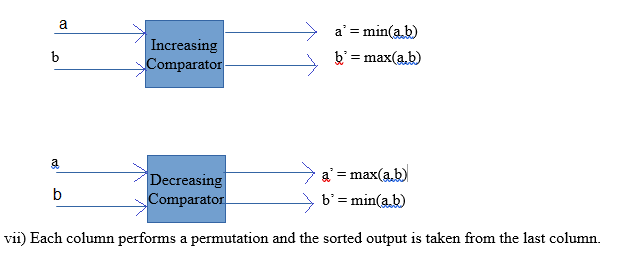vii) Each column performs a permutation and the sorted output is taken from the last column.Fig: Schematic diagram of a sorting network

Q5) b) Explain Single Shortest Path Algorithm with suitable Example.

Single Source Shortest Path

1. i) A traveler wishes to find a shortest distance between New Delhi and Visakhapatnam, in a road map of India in which distance between pair of adjacent road intersections are marked in kilometers.
2. ii) One possible solution is to find all possible routes between New Delhi and Visakhapatnam, and find the distance of each route and take the shortest.

iii) There are many possible routes between given cities. It is difficult to enumerate all of them.

1. iv) Some of these routes are not worth considering. For example, route through Cinnai, since it is about 800KM out of the way.
2. iv) The problem can be solved efficiently, by modeling the given road map as a graph and find the shortest route between given pair of cites as suitable (single source shortest paths) graph problem.

Graph Representation of the road map: The intersections between the roads are denoted as vertices and road joining them as edge in the graph representation of the given road map.

1. i) Single Source Shortest Path problem: Given a graph G = (V, E), we want to find the shortest path from given source to every vertex in the graph.
2. ii) The problem is solved efficiently by Dijkstra using greedy strategy. The algorithm is known as Dijkstra’s algorithm.

iii) The method works by maintaining a set S of vertices for which shortest path from source v0 is found. Initially, S is empty and shortest distance to each vertex from source is infinity.

1. iv) The algorithm repeatedly selects a vertex viin V – S to which path form source is shorter than other vertices in V-S, adds to S, and estimates the shortest distance to other vertices from vi.

Algorithm Dijkstra_shortest_path(G, v_0)

Step 1: for each vertex vi in V do
Step 2:dist[vi} = infinity
Step 3: distance[v0] = 0;
Step 4:for each vertex v_i in V do
Step 5: insert(Q, vi)
Step 6: S = empty
Step 7: while (Q not empty) do
Step 8: vi = Min(Q)
Step 9: for each vertex vj adjacent to vi do
Step 10: distance[vj] = Minimum (distance[vj], distance[vi] + w[vi,vj])

• Consider the Following Graph• The following sequence of figures shows the way of finding the shortest path from source vertex v0.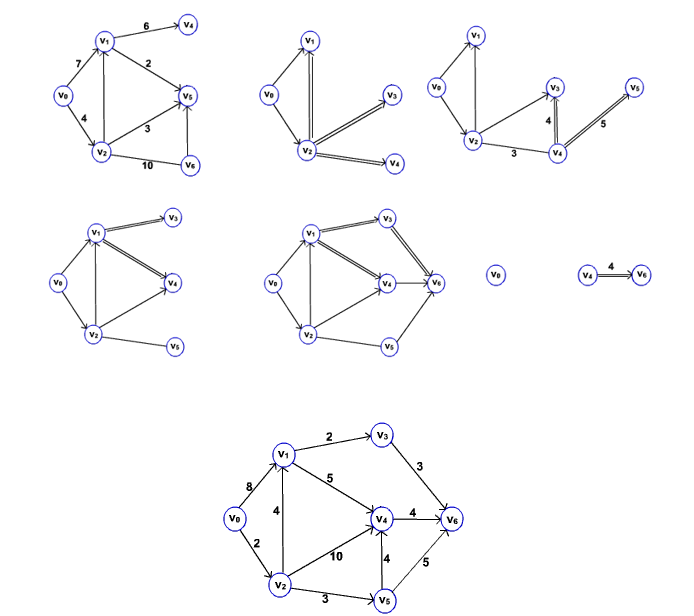• The event queue in Dijkstra’s algorithm can be implemented using array or heap.

All pairs shortest path

• Problem: In a given weighted directed graph find the shortest path between every pair of vertices.
• This problem can be solved by repeatedly invoking the single source shortest path algorithm for each vertex as a source.
• Another way of solving the problem is using dynamic programming technique.

Q6) a) Describe Different Techniques of Latency Hiding.

1. i) Generally the higher latency is due to the shared resources in a network, that too shared memory. The following methods can be used to hide the latency.
2. a) Perfecting Instructions.
3. b) Use of coherant cache.
4. c) Use of relaxed memory consistency model.
5. d) Communication.

1. a) Perfecting Instructions.
2. i) The instructions that are to be executed in next clock cycles are perfected. This can be either hardware of software controlled.
3. ii) The hardware controlled perfecting is achieved by using long cache lines or instruction look ahead. Software controlled perfecting executes the perfecting instructions explicitely to move data close to the processor before it is needed.

b)Use of Coherant Cache.

1. i) Multiple caches are used to store the data. The cache coherance has two levels.
2. Every write operation occurs instantaneously.
3. All processor present in the network see the same sequence of changes in the data.
4. ii) The cache coherenace is mainly used to manage the conflicts between the cache and main memory. The access to cache memory is faster than main memory. This reduces / hides the latency in case of fetching any instruction or data.

1. c) Use of relaxed memory consistency model.
2. i) In relexed or release model, the synchronization accessor of data in the program are idenfified. They are classified as locks or unlocks. This provides flexibility and fast access.
3. ii) In multiprocessor architecture, relaxed memory model is implemented by buffering the write operations and allowing the read operations.

1. d) Communication.
2. i) In case of communication, a block of data is transferred rather than a single data; Generating a communication before it is actually needed helps to reduce the latency. This is known as pre-communication.

Q6) b) How latency hiding is different than latency reduction?

Memory Latency Hiding: Finding something else to do while waiting for memory.

Memory Latency Reduction: Reducing time operations must wait for memory.

Latency Hiding in Covered Architectures:

1. Superscalar (Hiding cache miss.)
2. While waiting execute other instructions.
3. Can only cover part of miss latency.

2. While waiting execute other threads.
3. Can cover full latency.

iii. Requires effort to parallelize code.

1. Parallel code possibly less efficient.

1. Multiprocessor
2. Latency problem worse due to coherence hardware and distributed memory.

Memory Latency Reduction Approaches

1. Vector Processors
2. ISA and hardware eciently access regularly arranged data.

ii.Commercially available for many years.

1. Software Prefetch
2. Compiler or programmer fetch data in advance.
3. Available in some ISAs.

1. Hardware Prefetch
2. Hardware fetches data in advance by guessing access patterns.

1. Integrated Processor /DRAM

i.Reduce latency by placing processor and memory on same chip.

1. Active Messages

i.Send operations to where data is located.

Q7) a) Write Short Notes on:

1. Petascale Computing.
2. Nano Technology.
3. Power aware Computing.

1. Petscale Computing.

In computing, petascale refers to a computer system capable of reaching performance in excess of one petaflops, i.e. one quadrillion floating point operations per second. The standard benchmark tool is LINPACK and Top500.org is the organization which tracks the fastest supercomputers. Some uniquely specialized petascale computers do not rank on the Top500 list since they cannot run LINPACK. This makes comparisons to ordinary supercomputers hard.Petascale can also refer to very large storage systems where the capacity exceeds one petabyte (PB).Petascale computing is being used to do advanced computations in fields such as weather and climate simulation, nuclear simulations, cosmology, quantum chemistry, lower-level organism brain simulation, and fusion science.

1. Nano Technology.
2. i) Nanotechnology is a technology that manipulates matter on an atomic molecular and super molecular scale. It is used in fabrication of microscale products.
3. ii) The size of the matter considered would be in a range of 1 to 100 nano meters (10-9 m).

iii) Use of nanotechnology improves the performance of HPC. If HPC is used with multiscale optimization library using nanotechnology, then the reliability calculation can be accelerated.

1. iv) The computations in an HPC setup can be optimized using nanotechnology. Usage of nanotechnology will be more effective, when work distribution is done in different clusters.
2. v) The computation on a CUDA based framework will be more effective, when it is based along with nanotechnology and parallelization capabilities of CPU.

1. Power Aware Computing.
2. i) In high-performance systems, power-aware design techniques aim to maximize performance under power dissipation and power consumption constraints. Along with thsi, the low-power design techniques try to reduce power or energy consumption in portable equipment to meet a desired performance. All the power a system consumes eventually dissipates and transforms into heat.

Q7) b) Elucidate Thread Organization in Detail.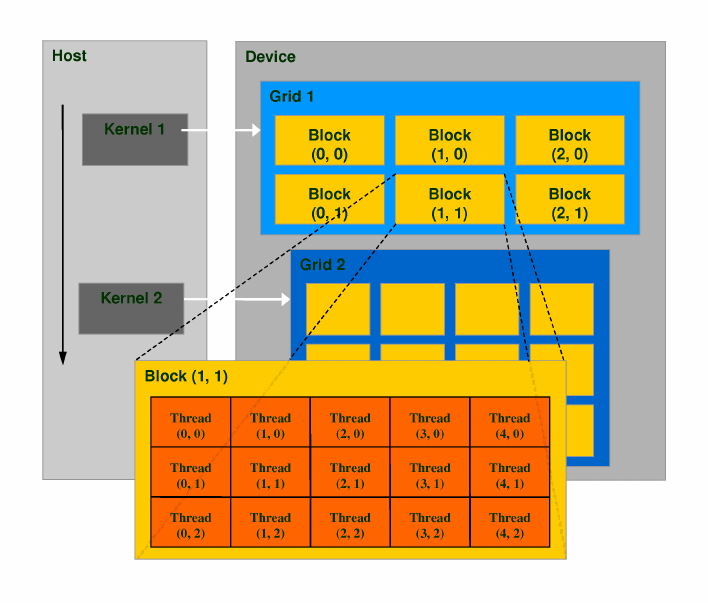Q8) a) Write Short Notes on (Any Two)

1. Discrete Optimization Problem.
2. Parallel Best-First Search.
3. Quantum Computers.

1. Discrete Optimization Problem.
2. i) A discrete optimization problem is a tuple (S,f), where S is set of all solutions satisfy the given constraints. S can be either finite or countably infinite. S is called the set of possible or feasible solutions.
3. ii) f is a function from S to the set of real numbers R, represented as, f:S —> R

iii) It is called as cost function.

1. iv) The main task in discrete optimization problem is to find optimal solution xopt from the set of feasible solutions S, such that

∀ x ∈ S, f(xopt)  <= f(x)

1. v) Discrete Optimization Problem can be solved using following methods.
2. Brute force Search.
3. Maximization-Minimization.

1. Parallel Best First Search.
2. i) Best First Search (BFS) algorithm can be used to search both trees and graphs. This algorithm uses heuristics to select the portion of search space.
3. ii) The nodes which are near to result or seem to give a result are assigned with smaller heuristic values and nodes away from the result are assigned with larger heuristic method.

iii) This algorithm maintains two lists: an open list and a closed list. Initially, the first node is placed on the open list. The algorithm uses a predefined heuristic evaluation function. This function measures how likely the node can get closer to result.

1. iv) The open list is sorted as per this function.
2. v) In each step, the most promising node from the open list is removed. If it is the required resulting node, then the algorithm is terminated.

1. Quantum Computers.
2. i) Quantum is the minimum amount of any physical entity which is involved in any communication.
3. ii) The computer which is designed by using the principles of quantum physics is called quantum computer.

iii) A quantum computer stores the information using special types of bits called quantum bit represented as |0> and | 1 >.

1. iv) This increases the flexibility of the computations. It performs the calculations based on the laws of quantum physics.
2. v) The quantum bits are implemented using the two energy levels of an atom. An excited state represents | 1> and a ground state represents | 0>.
3. vi) Quantum gates are used to perform operations on the data. They are very similar to the traditional logical gates.

vii) Since the quantum gates are reversible, we can generate the original input from the obtained output as well.

viii) A quantum computer has the power of atoms to perform any operation. It has a capability of processing millions of operations parallely.

Q8) b) Intricate sorting issues in Parallel Computers.

1. i) The major task in the Parallel Algorithms is to Distribute the elements to be sorted onto the available processes. Following are the issues that are to be addressed while distributing.

1 .Where the input and output sequences are stored.

1. i) In case of parallel algorithm, the data may be present in one process or may be it is distributed throughout the processes. Wherever sorting is a part of any other algorithm, it is better to distribute the data among processes.
2. ii) To distribute the sorted output sequence among the processes, the processes must be enumerated. This enumeration is used to specify the order of the data.

iii) For example, the Process P1 comes before Process P2 in enumeration, then all the elements in Process P1 comes before all the elements in Process P2 in the sorted sequence.

1. iv) This enumeration mainly depends on the type on interconnection network.

1. How Comparisons are Performed.
2. i) In case of sequential algorithms, all the data that is to be compared is present on only one process, whereas in case of parallel algorithms, data is distributed to n number of processes. Hence there can be two cases in case of how comparisons are performed.

Case 1: Each Process Has Only One Element

1. i) Let Process P1 contains element having value ‘1’ and Process P2 contains element having value as ‘2’. Let process P1 comes before P2. To arrange two elements in ascending order, first thing to do is send the element from one process to another and compare them in the process.
2. ii) Here P1 keeps smaller value whereas P2 keeps larger.

Iii) Hence both the process send the data to one another. So both the process can perform compare operation.1. iv) Process P1 and P2 both contains value 1 and 2 after sending Data to each other. Now because of enumeration, Process P1 comes before Process P2. Hence Process P1 Performs min(1,2) compare operation i.e. Minimum between two elements gets selected. In this case it is 1.
2. v) Similiarily, Process P2 performs max(1,2) compare opertion i.e. Maximum between two elements is selected.In this case it is 2.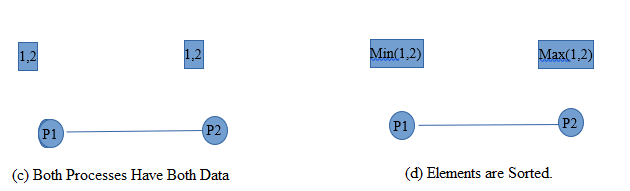1. v) If P1 and P2 are neighbours in the interconnection network and communication is bidirectional, then communication cost is ts + tw.

Case 2: Each Process Has More Than one Element.

1. i) When there is large sequence is to be sorted and number of processes available in less then each process stores more than one element in its memory. If there are n elements to be sorted and number of processes available is p, then n/p elements are allotted to each process.
2. ii) In this case also, consider the example of two processes P1 and P2. Let us consider that P1 contains elements (2,5,8,9) and P2 contains (1,3,6,7).

iii) Similar to Case 1, in this case also both the processes sends the data to each other using communication network.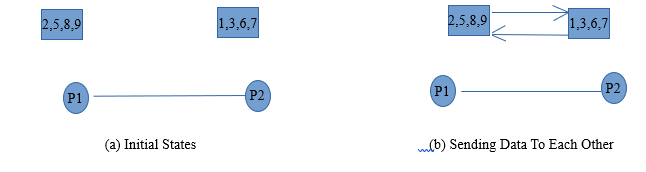1. iv) Again Both the process have all the data of each other similar to case 1.
2. v) On next step Data is sorted and again minimum and maximum operations are performed. Minimum half is kept in P1 and Maximum in P2.
3. vi) After that two process are merged to get output.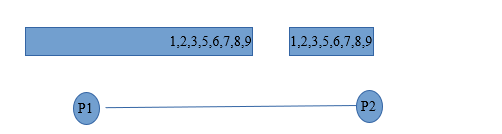(c) Both Processes Have Both Data and Sorted Data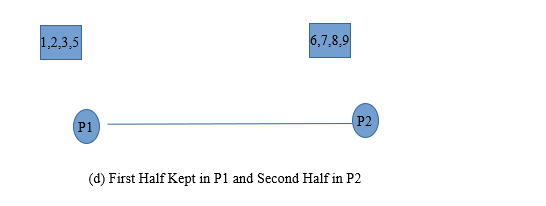vii) Complexity: O(ts + tw n/p).

n/p since each process contains n/p and communication is bidirectional.

This site uses Akismet to reduce spam. Learn how your comment data is processed.